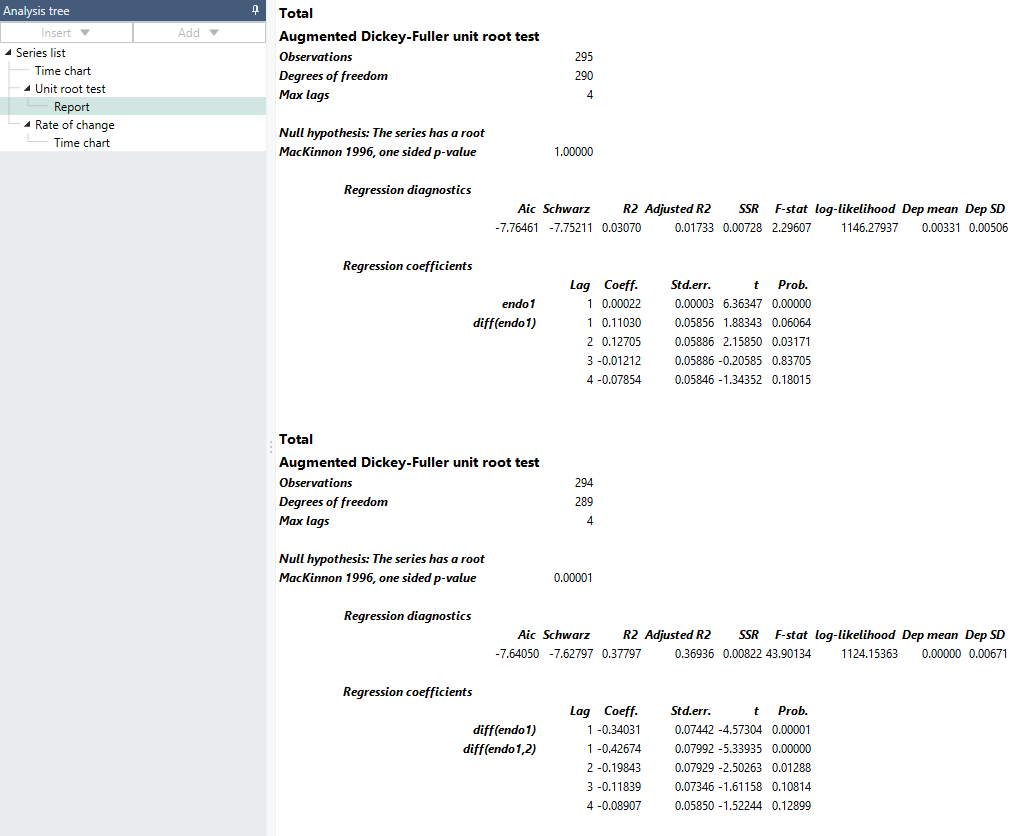# Overview

The Unit Root Test provides you with a tool to test if a series is non-stationary. More specifically, it performs an Augmented Dickey-Fuller (ADF) test of the null hypothesis that a time series has a unite root, which will violate the underlying assumptions in many statistical models. The analysis produces a report in which you can read and interpret results from the statistical test.

# Estimation model

If a unit root is present in the system, the variance may be infinite overall and the data can be difficult to use in further analysis. The ADF test regression equation has the form:

$\mathrm{\Delta }{y}_{t}=\alpha {y}_{t}+\sum _{i=1}^{p}{\beta }_{i}\mathrm{\Delta }{y}_{t-i}+\gamma c+\delta t+{e}_{t}$

$\alpha$ is the coefficient of the endogenous variable y on level form and is used for the actual test. Viewed as an autoregressive process, AR(p), the $\beta$-coefficients are for the p lags of y on difference form, $\mathrm{\Delta }y$. The ADF test (Dickey & Fuller, 1979) introduced lagged differenced variables to whiten the residuals, e, in the estimation. The lag length, p, should be chosen carefully observing the change in information criterion and should apply to the AR-model regardless of whether it has a unit root. (Sometimes t-values are used as criteria instead.) If an AR(1) process is assumed, all $\mathrm{\Delta }y$-terms can be omitted by setting both min and max lag to zero, given that e is still trusted to be approximately white noise. $\gamma$ is the coefficient for the constant term and  for the linear trend.

If the series is assumed to be integrated, it can be differenced by checking the diff box. Note that trend and constant terms are not affected by this.

The null hypothesis is that the series has a unit root, so that  is equal to zero, or:

${H}_{0}:\alpha =0$ ${H}_{1}:\alpha <0$

Evaluation is done with t-values from the sample statistics, ${t}_{\alpha }=\stackrel{^}{\alpha }/{\stackrel{^}{\sigma }}_{\alpha }$ after OLS estimation. Because the t-values of the ADF estimator are non-standard both in sample and asymptotically, special tables and response surface calculations from MacKinnon 1996 are used (with permission) to calculate the p-value. The settings for the deterministic variables are in a drop-down box. The MacKinnon calculations will adjust to this (and to sample size) automatically but cannot account for other deterministic (or exogenous) variables, so MB does not allow them in the ADF test.

# Report

The Unit Root Test automatically generates a report, which includes a variety of statistical information.# Settings### Start Lag/End Lag

To perform the analysis, you must specify which number of lags that will be included in the test. If you change 'Start lag' to 1 and 'End lag' to 3, it will include lags of 1, 2 and 3 in the test. It is also possible to run the test in a classical Dickey Fuller setup, without any lagged differences, by setting both min and max lag to zero.

### Include

Select if you want to include this series in the analysis.

### Diff

By selecting Diff, the first order differences of the series will be calculated. The result will then be converted back to levels. First order of differences means that the series is transformed to "Change in value" (one observation) while expressing the result in levels.

### Deterministic variables

Select if you want to include any deterministic variables in the equation, intercept or intercept and linear trend.

# Examples

US GDP

In this example, we used the Unit Root Test on the U.S. GDP, expressed in logarithm. One version of the series has been expressed in differentials, to see how results would differ.